Next: 6. Conclusion and Outlook Up: 5.4 Three-Dimensional Simulation Results Previous: 5.4.2 Local oxidation with

## 5.4.3 Polybuffered LOCOS

The last example to be presented is a polybufferd LOCOS. It is calculated again with a floating nitride mask, but now residing on the top of a polysilicon layer. In this case not only the silicon layer shows a high oxidation ratio but also the polysilicon layer and therefore both are calculated with the same equations for the oxidation model introduced in the first example (Section 5.4.1).

To show the abilities of the model the equations are extended with an in-diffusion segregation equation introduced in Chapter 4.8.2 including the field enhancement factor of Chapter 2.1:= divDeff(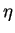)gradC-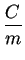grad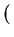m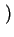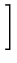(5.29)

with
 Deff() = D() .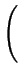1 +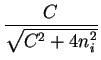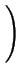(5.30)

With these equations a background doping of boron with a constant concentration of 1015situated inside the silicon layer is calculated. The diffusivity of the dopant depends on the local concentration as well as on the underlying material leading to another nonlinearity within the total system.

The developed differential equation systems have now three unknowns in the nitride mask (X,Y,Z), five unknowns in the polysilicon layer (X,Y,Z,O2,SiO2) and six unknowns in the silicon layer (X,Y,Z,O2,SiO2,Boron), each of which is calculated on a separate grid. The layers are connected to each other along the interface boundaries. The example is calculated at a temperature of 1000oC as well as 1100oC to show the differences of oxide growth especially in the corners of the oxidizing layers.

To solve this large example the grid was adapted towards the interface between (poly-) silicon and silicon dioxide. However, because of the enormous rank of the resulting global system matrix the quality of the grid was comparably poor for a three-dimensional problem. It was calculated with about 9000 points (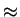28000 elements) for the silicon layer, approximately 4500 nodes (13000 elements) for the polysilicon layer and about 1000 nodes (2000 elements) for the nitride mask. All together the memory consumption of this example holds at 150MB with a calculation duration of 1 hour and 45 minutes.

Fig. 5.15 shows the calculated result of the generated silicon dioxide and its influence concerning volume dilatation at a temperature of 1000oC where Fig. 5.16 shows the result at 1100oC. As in the two-dimensional case the lower temperature leads to a thinning at the corners whereas the high temperature calculation shows the rounded corners as expected. Again the calculation were made using a surface strain in dependence of temperature. The higher the temperature the more viscosity is assumed for the material and the more strain is imprinted at the surface. Together these effects lead to a smoother surface as well as the typical rounded areas at the inner interface boundaries.

Fig. 5.17 shows the distribution of polysilicon, silicon and the generated oxide of the high temperature calculation. In the center of the polysilicon layer the oxidation is still not finished which leads to the remaining deformations of the nitride mask. If the calculation time is increased the polysilicon layer would completely be oxidized an the deformations of the nitride mask are reduced again.

Figure 5.15: Three-dimensional polysilicon growth at a temperature of 1000oC with a detail picture of a oxidizing corner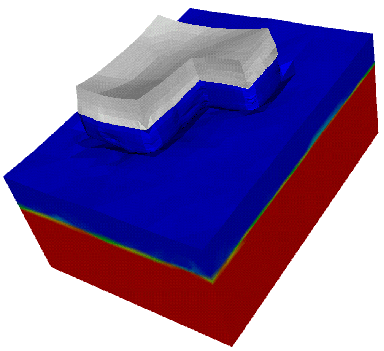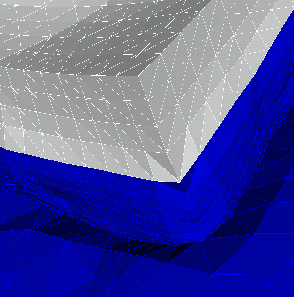Figure 5.16: Three-dimensional polysilicon growth at a temperature of 1100oC with a detail picture of a oxidizing corner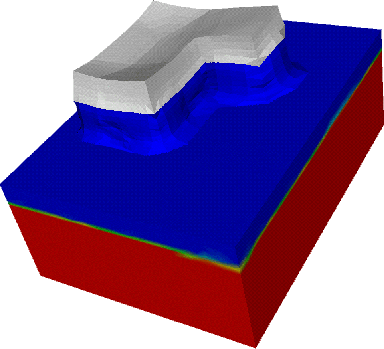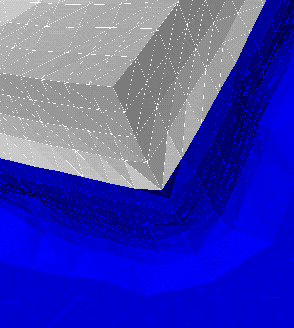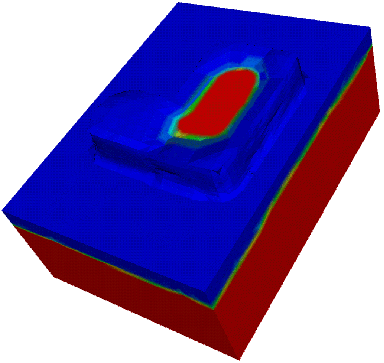Finally, Fig. 5.18 shows a post-processed cut of the low temperature oxidation and Fig. 5.19 the distribution of Boron resulting of the high temperature oxidation. As expected, the boron concentration shows a segregation behavior due to the material interface between silicon and silicon dioxide.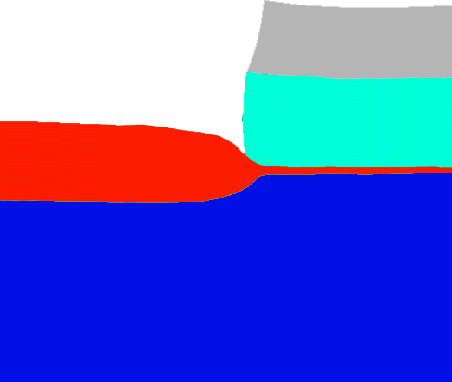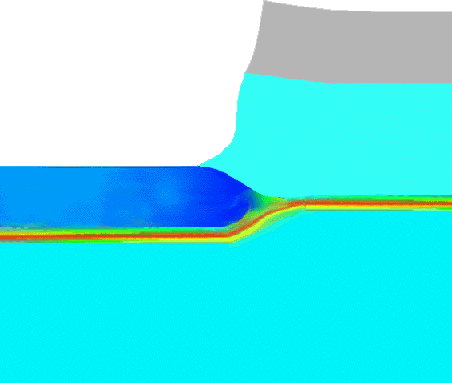Next: 6. Conclusion and Outlook Up: 5.4 Three-Dimensional Simulation Results Previous: 5.4.2 Local oxidation with